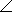# Electrical Engineering - RC Circuits - Discussion

### Discussion :: RC Circuits - General Questions (Q.No.21)

21.

A 12 kresistor is in series with a 0.02F capacitor across a 1.2 kHz ac source. If the current is expressed in polar form as I = 0.30° mA, what is the source voltage expressed in polar form?

 [A]. 45.6 V [B]. 411.3 V [C]. 0.411 V [D]. 4.11 V

Explanation:

No answer description available for this question.

 Selva said: (Mar 9, 2012) FROM OHM'S LAW V=IZ Z=Sqr(R^2+Xc^2) Xc=(1/(2*pi*f*C)) Xc=(10^3/(2*pi*1.2*0.02)) =(1000/0.15)=6666.67 ohms Z=sqr(12000^2+6666.67^2)=13727.508 ohms V=IZ=13727.508*0.3 mA =4.11 volts

 Chester said: (Jul 26, 2017) Answer should be in mV.

 Nomiki said: (Jun 11, 2019) The answer should be in Volts.

 Rimah said: (Sep 13, 2019) Why we have to the square the resistance and capacitance values?